# JP4429888B2 - Angle detection sensor with compensation function - Google Patents

## Info

Publication number
JP4429888B2
JP4429888B2 JP2004364504A JP2004364504A JP4429888B2 JP 4429888 B2 JP4429888 B2 JP 4429888B2 JP 2004364504 A JP2004364504 A JP 2004364504A JP 2004364504 A JP2004364504 A JP 2004364504A JP 4429888 B2 JP4429888 B2 JP 4429888B2
Authority
JP
Japan
Prior art keywords
compensation
signal
angle
output
distortion
Prior art date
Legal status (The legal status is an assumption and is not a legal conclusion. Google has not performed a legal analysis and makes no representation as to the accuracy of the status listed.)
Active
Application number
JP2004364504A
Other languages
Japanese (ja)
Other versions
JP2006170837A (en
Inventor

Original Assignee
アルプス電気株式会社
Priority date (The priority date is an assumption and is not a legal conclusion. Google has not performed a legal analysis and makes no representation as to the accuracy of the date listed.)
Filing date
Publication date
Application filed by アルプス電気株式会社 filed Critical アルプス電気株式会社
Priority to JP2004364504A priority Critical patent/JP4429888B2/en
Publication of JP2006170837A publication Critical patent/JP2006170837A/en
Application granted granted Critical
Publication of JP4429888B2 publication Critical patent/JP4429888B2/en
Application status is Active legal-status Critical
Anticipated expiration legal-status Critical

## Images

•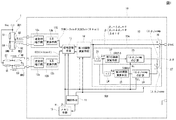•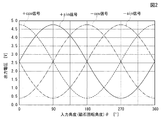•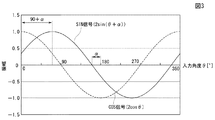•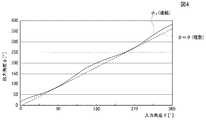•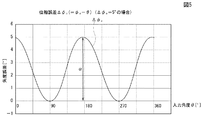•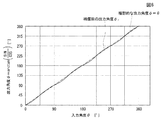•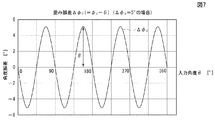•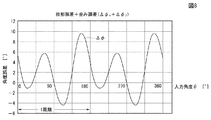•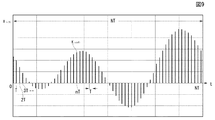•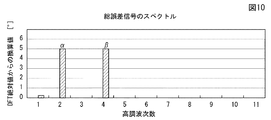•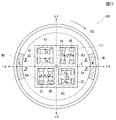## Description

The present invention relates to an angle detection sensor using a magnetoresistive effect element typified by a GMR element, and is particularly detected when a signal output from the magnetoresistive effect element includes a phase error or a distortion error. The present invention relates to an angle detection sensor having a compensation function capable of increasing the detection accuracy of output angles.

Detection of an output angle of a steering wheel of an automobile is performed using a wheel that rotates in synchronization with a rotating member such as a steering shaft and an angle detection sensor. The sensor portion of the angle detection sensor employs a magnetoresistive effect element that senses magnetism and outputs an output signal. As a prior art document of an angle detection sensor using such a magnetoresistive effect element, for example, The following patent documents 1, 2, 3, and 4 exist.

FIG. 11 is a plan view showing the configuration of the angle detection sensor 100. The angle detection sensor 100 is provided with a wheel 102 that rotates with respect to the rotation center O and a package 101 therein.

In the package 101, four chip substrates (wafers) K1, K2, K3, and K4 are provided at positions symmetrical to the rotation center O (positions shifted by 90 ° around the rotation center O). Yes. One chip substrate basically has a structure in which an exchange bias layer (antiferromagnetic layer), a fixed layer (pinned layer), a nonmagnetic layer, and a free layer (free magnetic layer) are stacked. Two GMR elements (indicated individually as G1 to G8) as magnetoresistive elements are provided.

That is, the chip substrate K1 is provided with GMR elements G1 and G2, the chip substrate K2 is provided with GMR elements G3 and G4, the chip substrate K3 is provided with GMR elements G5 and G6, and the chip substrate K4 is provided with GMR elements G7 and G8 are provided. The GMR elements G1 to G8 mounted on the chip substrates K1 to K4 are parallel to each other with the GMR element G1 and the GMR element G4 being connected in series and the GMR element G3 and the GMR element G2 being connected in series. A first bridge circuit is configured by connection. Similarly, the GMR element G5 and the GMR element G8 are connected in series, and the GMR element G7 and the GMR element G6 are connected in series to form a second bridge circuit (FIG. 1). reference).

Magnets M1 and M2 are fixed to the inner surface of the wheel 102. One magnet M1 is fixed with the N pole directed to the rotation center O and the other magnet M2 is fixed with the S pole directed to the rotation center O, and a fixed external portion is provided between the magnet M1 and the magnet M2. A magnetic field H is generated.

When the rotating member as the object to be measured rotates and the wheel 102 is rotated, the magnets M1 and M2 circulate around the package 101. At this time, the magnetization direction of the free layer of each of the GMR elements G1 to G8 is changed according to the external magnetic field H. As a result, the resistance values of the GMR elements G1 to G8 change according to the angle formed by the magnetization direction of the free layer and the magnetization direction of the fixed layer. A −sin signal is output, and at the same time, the second bridge circuit outputs a + cos signal and a −cos signal whose phases are shifted by 90 ° from the ± sin signal of the first bridge circuit.

Of these four signals, the control unit differentially amplifies the + sin signal and the −sin signal to generate a SIN signal (sine wave signal), and differentially amplifies the + cos signal and the −cos signal. To generate a COS signal (cosine wave signal). Next, the control unit calculates a tangent value (tan) from the SIN signal (sine wave signal) and the COS signal (cosine wave signal), and further calculates an arc tangent value (arctan), thereby obtaining the rotation member. The output angle can be detected.
JP 2002-303536 A JP 2000-35470 A JP 2003-106866 A JP 2003-66127 A

In the angle detection sensor 100, in order to detect the rotation angle of the rotating member with high accuracy, it is necessary to maintain the phase difference of 90 ° between the sine wave signal and the cosine wave signal with high accuracy. For this purpose, the magnetization directions (magnetization directions) e of the fixed layers of the two GMR elements provided on the same chip substrate are manufactured in the same direction. For example, the magnetization direction of the chip substrate K1 When e is the + Y direction, the magnetization direction e of the chip substrate K2 is the -Y direction, the magnetization direction e of the chip substrate K3 is the + X direction, and the magnetization direction e of the chip substrate K4 is the -X direction. Therefore, it is necessary to mount so that the magnetization directions e are shifted from each other at 90 ° intervals with high accuracy.

However, since the magnetization direction e of the fixed layers of the GMR elements G1 to G8 cannot be visually confirmed, the chip substrates K1 to K4 are mounted on the package so that the magnetization direction e is accurately shifted by 90 °. When the 90 ° cannot be set accurately, the phase error α occurs as 90 ° ± α, and the rotation angle (output angle) of the rotating member cannot be detected with high accuracy. There was a problem.

Further, when each chip substrate is cut out with high accuracy and the magnetization direction e of the GMR elements G1 to G8 is formed with high accuracy parallel to one side of the chip substrate, for example, an image recognition device or the like By making full use of a device for correcting the mounting angle and mounting the chip substrates at 90 ° on the package, the magnetization direction e can be accurately shifted by 90 °. In this case, the manufacture of the chip substrate is possible. There is a problem that the cost is likely to rise, and the assembling process at the time of mounting becomes complicated, resulting in an increase in assembling time and assembling cost.

On the other hand, in the angle detection sensor 100, it is ideal that the output angle φ is output in proportion to the input angle (magnet rotation angle) θ that the wheel 102 rotates. Becomes a distortion error in which a sinusoidal signal is superimposed on a straight line that changes with a linear function, and the output angle φ may not be exactly proportional to the input angle θ (see FIG. 6).

It is known that the cause of such a distortion error is caused by a resistance value distortion peculiar to the GMR element, and each of the four signal waveforms as the output of the angle detection sensor 100 has such a distortion error. Therefore, a distortion error also occurs in the SIN signal and the COS signal. As a result, the calculation of the tangent value (tan) and arc tangent value (arctan) is also affected by the distortion error. There is a problem that the accuracy of the output angle φ cannot be increased.

The phase error α and the distortion error β can be approximated by a predetermined function, and if the output angle φ output from the angle detection sensor 100 can be compensated for each angle using the approximate function, the output The accuracy of the angle φ can be greatly increased. However, the correction coefficient constituting the predetermined function cannot be easily calculated, and the above Patent Documents 1, 2, 3 and 4 do not describe a method for obtaining the correction coefficient.

The present invention is to solve the above-described conventional problems, and the four signals output from the magnetoresistive effect element include an error signal due to the phase error and / or distortion error as described above. Even in this case, an object of the present invention is to provide an angle detection sensor having a compensation function that can improve the detection accuracy of the output angle detected by reducing these effects.

It is another object of the present invention to provide an angle detection sensor having a compensation function that can easily calculate a phase compensation value and a distortion compensation value that approximately indicate a phase error α and a distortion error β.

The present invention provides a sensor unit that generates a rotating magnetic field according to rotation given to an object to be measured and senses the rotating magnetic field and outputs a plurality of output signals according to an input angle of the rotation, and the output signal In an angle detection sensor comprising: a signal processing unit that calculates an output angle as an angle at which the object to be measured is rotated from
The signal processing unit includes a signal conversion unit that generates two types of signals having a predetermined phase difference from the output signal, and a first that calculates an output angle of the device under test before compensation from the two types of signals. Function calculating means, a compensation means for calculating a compensation value for removing an error signal included in the output angle before compensation, and a compensation coefficient used when the compensation means calculates the compensation value. a memory means for storing Te, wherein a control unit that reads the compensation coefficient stored in the memory means given to the compensating means, is provided, et al is,
The error signal is a distortion error generated based on characteristics of the sensor unit, and the compensation unit is provided with a distortion compensation unit that generates a distortion compensation value for reducing the distortion error. To do.

According to another aspect of the present invention, there is provided a sensor unit that generates a rotating magnetic field according to the rotation given to the object to be measured, senses the rotating magnetic field, and outputs a plurality of output signals according to the rotation input angle; In an angle detection sensor comprising: a signal processing unit that calculates, as an output angle, an angle at which the measured object rotates from a signal,
The signal processing unit includes a signal conversion unit that generates two types of signals having a predetermined phase difference from the output signal, and a first that calculates an output angle of the device under test before compensation from the two types of signals. Function calculating means, a compensation means for calculating a compensation value for removing an error signal included in the output angle before compensation, and a compensation coefficient used when the compensation means calculates the compensation value. A memory means for storing and a controller for reading the compensation coefficient stored in the memory means and providing the compensation means to the compensation means,
The error signal is a phase error generated due to exceeding the predetermined phase difference existing between the two types of signals and a distortion error generated based on the characteristics of the sensor unit. in which the distortion compensation unit for generating a distortion compensation value for reducing the phase compensating portion and the distortion error for generating a phase compensation value for reducing the phase error is eclipsed set.

In the above, it is preferable that the memory means stores a value obtained by performing a discrete Fourier transform on data obtained by sampling an error signal included in the output angle before compensation as a compensation coefficient.

In the above, the compensation coefficient is at least one of a phase compensation coefficient and a distortion compensation coefficient.
In this case, the positive or negative sign given to the phase compensation coefficient can be determined by a real sign when the harmonics obtained by performing the discrete Fourier transform are represented by complex numbers.

For example, the phase compensation coefficient can be obtained from a second harmonic component, and the distortion compensation coefficient can be obtained from a fourth harmonic component .

In the present invention, it is possible to easily obtain a phase compensation value and a distortion correction value that enhance the accuracy of the output angle of the angle detection sensor.

Moreover, since it is only necessary to store the phase compensation coefficient, its sign, and the distortion compensation coefficient, the amount of data is small, and the readout time can be greatly shortened. Therefore, the output angle of the angle detection sensor can be detected in real time.

FIG. 1 is a block diagram showing the configuration of the angle detection sensor of the present invention, and FIG. 2 is a waveform diagram showing the relationship between four signals output from two sets of bridge circuits as an ideal case. An angle detection sensor described below detects an output angle of a rotating member such as a steering shaft of an automobile.

The angle detection sensor shown in FIG. 1 includes a sensor unit 1 and a signal processing unit 10 that processes an output signal output from the sensor unit 1. The sensor unit 1 is shown on the left side of the illustrated PP line, and the signal processing unit 10 is shown on the right side.

The configuration of the sensor unit 1 is the same as that described in the section “Background Art”. That is, as shown in FIG. 11, the sensor unit 1 includes a wheel 102 that is rotatably provided with respect to a rotation center O, and four chip substrates (wafers) K1, K2, K3, and K4 in the wheel 102. And a package 103 mounted thereon. The four chip substrates K <b> 1 to K <b> 4 are provided in positions that are symmetrical with respect to the rotation center O in the package 103, that is, positions that are shifted from each other by 90 ° around the rotation center O.

One chip substrate has a structure in which an exchange bias layer (antiferromagnetic layer), a fixed layer (pinned layer), a nonmagnetic layer, and a free layer (free magnetic layer) are stacked (not shown). Two GMR elements (indicated individually as G1 to G8) are provided as magnetoresistive effect elements.

The chip substrate is subjected to an external magnetic field in a state where a plurality of GMR elements are formed on a single large substrate, and the magnetization direction (magnetization direction) of the fixed layer is aligned in a certain direction. Since it is divided into chip substrates K1 to K4, the magnetization directions of the fixed layers of the two GMR elements provided on one chip substrate are the same. The chip substrates K1 to K4 are fixed in the package 101 so that the magnetization directions have a relationship of approximately 90 ° between adjacent substrates. The 90 ° relationship is preferably accurate. However, the 90 ° relationship is not necessarily required with a phase compensation circuit described later.

The GMR elements G1 to G8 mounted on the chip substrates K1 to K4 constitute a first bridge circuit WB1 and a second bridge circuit WB2. As shown in FIG. 1, the first bridge circuit WB1 is composed of chip substrates K1 and GMR elements G1, G2 and G3, G4 mounted on the chip substrate K2 and arranged symmetrically with respect to the rotation center O. ing. That is, the first bridge circuit WB1 is formed by connecting a circuit in which the GMR element G1 and the GMR element G4 are connected in series and a circuit in which the GMR element G3 and the GMR element G2 are connected in series. Has been. Similarly, the second bridge circuit WB2 is composed of a chip substrate K3 provided symmetrically with respect to the rotation center O and GMR elements G5, G6 and G7, G8 mounted on the chip substrate K4. The second bridge circuit WB2 is formed by connecting a circuit in which the GMR element G5 and the GMR element G8 are connected in series and a circuit in which the GMR element G7 and the GMR element G6 are connected in series to each other. Yes.

One end of the first bridge circuit WB1 and the second bridge circuit WB2 connected in parallel is connected to the power source Vcc, and the other end is grounded to the ground GND.

The wheel 102 and a rotating member (steering shaft or the like), which is an object to be measured, are connected via, for example, a gear, and the wheel 102 is configured to be rotated according to the rotation of the rotating member. Therefore, when the rotating member is rotated, the wheel 102 is rotated, so that the magnets M1 and M2 can go around the package 101.

At this time, the external magnetic field H generated between the magnets M1 and M2 gives a rotating magnetic field to the GMR elements G1 to G8 in the package 101, so that the free layer forming the GMR elements G1 to G8 The direction of magnetization is changed. As a result, the resistance values of the GMR elements G1 to G8 change according to the angle formed by the magnetization direction of the free layer and the magnetization direction of the fixed layer. Therefore, two sine wave-like phases that are 180 ° different from each other from the connection portion between the GMR element G3 and the GMR element G2 constituting the first bridge circuit WB1 and the connection portion between the GMR element G1 and the GMR element G4. A signal is output. At the same time, there are two sinusoidal two phases that are 180 ° different from each other in the connection portion between the GMR element G7 and the GMR element G6 and the connection portion between the GMR element G5 and the GMR element G8 constituting the second bridge circuit WB2. A signal is output.

However, the chip substrates K1 and K2, which are arranged symmetrically with respect to the rotation center O, and the chip substrates K3 and K4 which are arranged symmetrically with respect to the rotation center are arranged at positions that are approximately 90 ° different from the rotation center O. Therefore, if the two signals output from the first bridge circuit WB1 are the + sin signal and the −sin signal, the two signals output from the second bridge circuit WB2 are the + cos signal and the −cos signal. (See FIG. 2).

As shown in this embodiment, for example, when the wheel 102 rotates in the clockwise direction, a sinusoidal signal output from the connection portion between the GMR element G3 and the GMR element G2 of the first bridge circuit WB1 is + sin. As a signal, a -sin signal is output from the connecting portion between the GMR element G1 and the GMR element G4. At this time, a + cos signal is output from the connection between the GMR element G7 and GMR element G6 of the second bridge circuit WB2, and a -cos signal is output from the connection between the GMR element G5 and GMR element G8. become.

The signal processing unit 10 mainly includes a control unit 11, a first signal conversion unit 12A and a second signal conversion unit 12B, a signal adjustment unit 13, a first function calculation unit 14, a memory unit 15, a compensation unit 20, and the like. is doing.

The control means 11 is mainly composed of a CPU, and has a function of supervising a series of signal processing in the signal adjustment means 13, the first function calculation means 14, the compensation means 20, and the like. The first and second signal conversion means 12A and 12B have differential amplification means 12a and 12a and A / D conversion means 12b and 12b. The differential amplifying unit 12a of the first signal converting unit 12A differentially amplifies the two types of + sin signal and −sin signal output from the first bridge circuit WB1 and has a double amplitude. A sin signal is generated, and the A / D converter 12b converts the amplified signal into a SIN signal (digital signal) obtained by A / D conversion at a predetermined sampling period. Similarly, the differential amplifier 12a of the second signal converter 12B differentially amplifies the two types of + cos signal and −cos signal output from the second bridge circuit WB2, and doubles the amplitude. The A / D converter 12b converts the amplified signal into an A / D converted COS signal (digital signal).

Here, for example, A1, A2, B1 and B2 are amplitude coefficients, a1, a2, b1 and b2 are offset coefficients, the + sin signal is + A1 · sin θ + a1, the −sin signal is −A2 · sin θ−a2, and the + cos signal. Is expressed as + B1 · cos θ + b1 and the −cos signal is expressed as −B2 · cos θ−b2, the SIN signal generated by the first signal converting means 12A is (+ A1 · sin θ + a1) − (− A2 · sin θ−a2). ) = (A1 + A2) · sin θ + (a1 + a2). Similarly, the COS signal generated by the second signal converting means 12B is (+ B1 · cos θ + b1) − (− B2 · cos θ−b2) = (B1 + B2) · cos θ + (b1 + b2).

The signal adjusting means 13 has a function of performing offset adjustment and gain adjustment of the SIN signal and the COS signal, and matching the reference (0 point) and amount (amplitude amount) of the amplitude direction of both signals. That is, in the above example, the gain adjustment means that the amplitude coefficient of the SIN signal and the COS signal is set to the same value by setting A1 + A2 = B1 + B2, and the offset adjustment means that a1 + a2 = b1 + b2 = 0. This means that the deviation due to the bias voltage being superposed is eliminated so that the amplitude reference coincides with the origin position (0 point). Therefore, the SIN signal at this time is (A1 + A2) · sin θ, and the COS signal is (B1 + B2) · cos θ (where A1 + A2 = B1 + B2).

The first function calculation means 14 is software for calculating function values such as sin, cos, tan, tan −1 = arctan, sinh, cosh, exp, log, for example, a well-known CORDIC (Coordinate Rotation Digital Computer) algorithm. TAN processing for calculating a tangent value (tan = SIN signal / COS signal = sin θ / cos θ) by dividing the SIN signal by the COS signal, and the TAN And Atan processing for calculating the arc tangent value (arctan (sin θ / cos θ)) from the value obtained by the processing to obtain the output angle φ [°] before compensation of the object to be measured.

The compensation unit 20 in this embodiment includes a phase compensation unit 20A, a distortion compensation unit 20B, an addition unit 27, a subtraction unit 28, and the like. The phase compensation unit 20 </ b> A includes a first double angle unit 21, a second function calculation unit 22, and a phase compensation value generation unit 23. The distortion compensator 20B includes a second double angle means 24, a third function calculation means 25, and a distortion compensation value generation means 26. The second function calculation means 22 and the third function calculation means 25 are constituted by function calculation means using a CORDIC algorithm similar to the first function calculation means 14.

The first double angle means 21 has a function of doubling (2φ) the output angle φ before compensation obtained by the ATRAN processing of the first function calculation means 14 and outputting it. Further, the second double angle means 24 has a function of further doubling the output angle 2φ before compensation (doubled in total (4φ)) output by the first double angle means 21 by double. ing. The first and second double angle means 21 and 24 are composed of shift registers, for example, and shift data corresponding to the output angle φ before compensation expressed in binary notation to the left by 1 bit. Thus, 2φ (doubled) can be obtained, and further 4φ (fourfold) can be easily achieved by shifting left by 1 bit.

The second function calculating means 22 generates and outputs cos (2φ) from the signal 2φ output from the first double angle means 21 using the CORDIC algorithm. Similarly, the third function calculation means 25 generates sin (4φ) from the signal 4φ output from the second double angle means 24 using the CORDIC algorithm and outputs it.

The first function calculation unit 14, the second function calculation unit 22, and the third function calculation unit 25 share one function calculation unit, and each of the above calculations is performed by the function calculation unit. It may be configured to be used based on the control of the control means 11.

The phase compensation value generation means 23 reads out the cos (2φ) output from the second function calculation means 22, the phase compensation coefficient α 0 and the sign stored in the memory means 15, and reads out the phase compensation value ( Δφ α ) Comp is generated. The distortion compensation value generation means 26 reads out the sin (4φ) output from the third function calculation means 25 and the distortion compensation coefficient β 0 stored in the memory means 15 to read out the distortion compensation value (Δφ β ) Generate comp .

A specific method for obtaining the phase compensation value (Δφ α ) comp and the distortion compensation value (Δφ β ) comp will be described later.

The adding means 27 adds the phase compensation value (Δφ α ) comp and the distortion compensation value (Δφ β ) comp to give to the subtracting means 28. Then, the subtracting means 28 subtracts the phase compensation value (Δφ α ) comp and the distortion compensation value (Δφ β ) comp from the pre-compensation output angle φ obtained by the ATRAN processing of the first function computing means 14. Thus, it has a function of outputting a highly accurate output angle φ OUT . As another configuration replacing the adding means 27 and the subtracting means 28, one of the phase compensation value (Δφ α ) comp and the distortion compensation value (Δφ β ) comp is first subtracted from the output angle φ before the compensation. Then, the other may be subtracted.

As a result, the phase error α between the SIN signal and the COS signal can be eliminated, and the relationship between the two can be maintained at 90 ° with high accuracy, and at the same time, distortion based on resistance value distortion included in the output angle φ before compensation. The error β can be removed, and a highly accurate output angle φ OUT can be obtained.

Next, a specific method for obtaining the phase compensation value (Δφ α ) comp will be described.
FIG. 3 is a waveform diagram showing a case where a phase error is included between the SIN signal and the COS signal which are the outputs of the bridge circuit.

If the 90 ° relationship between the adjacent magnetization directions of the fixed layers constituting the GMR elements G1 to G8 provided on the chip substrates K1 to K4 is not maintained, the first and second signal conversion means The SIN signal and COS signal output from 12A and 12B, respectively, deviate from the original phase difference (90 °).

The output angle of the wheel 102, that is, the input angle input to the angle detection sensor 100 is θ, the phase error (shift amount from 90 °) at this time is α degrees, and the second bridge circuit WB2 The + cos signal output from is set to + cosθ, and the −cos signal is set to −cosθ. For convenience of explanation, the amplitude coefficients A1, A2, B1 and B2 are set to A1 = A2 = B1 = B2 = 1, and the offset coefficients a1, a2, b1 and b2 are set to a1 = a2 = b1 = b2 = 0. It is said. Even if it does in this way, since the same effect, ie, each said coefficient, will be erase | eliminated by the said signal adjustment means 13 or a TAN process after all, there is no problem in particular.

Based on + cos θ and −cos θ output from the second bridge circuit WB2, the + sin signal output from the first bridge circuit WB1 is expressed as + sin (θ + α), and the −sin signal is expressed as −sin (θ + α). be able to. Therefore, the SIN signal output from the first signal conversion unit 12A is + sin (θ + α) − (− sin (θ + α)) = 2sin (θ + α), and the COS signal output from the second signal conversion unit 12B. Is + cos θ − (− cos θ) = 2 cos θ, which is shown in FIG. In FIG. 3 and subsequent figures, a case where α = + 5 degrees is shown as an example of the phase error α.

The SIN signal and COS signal output from the first and second signal conversion means 12A and 12B are subjected to offset adjustment and gain adjustment in the signal adjustment means 13. Next, the tangent value (tan = SIN signal / COS signal) is calculated as 2 sin (θ + α) / 2 cos θ = sin (θ + α) / cos θ by the TAN processing of the first function calculating means 14. Although arctangent (arctan) are calculated by addition ATAN processing, and the output angle before compensation of the object to be measured which is the output of the first function operation unit 14 when the phase error alpha occurs phi alpha Then, φ α = arctan (sin (θ + α) / cos θ)).

FIG. 4 is a waveform diagram showing the output angle φ α before compensation after the Atan processing as a continuous function when phase error is included, and FIG. 5 detects the difference between the output angle φ α before compensation and the input angle θ. It is a waveform diagram shown as a phase error Δφ α (= φ α −θ).

In FIG. 4, there is a one-to-one correspondence between the input angle θ and the output angle φ α before compensation. However, the output angle φ α before compensation after the ATAAN processing has a form in which a trigonometric function is superimposed on a linear line (φ = θ) that is an ideal output angle.

When we calculated here by the difference between the phase detected by subtracting the input angle θ from the output angle phi alpha before the compensation the (phi alpha - [theta]) as detected phase error [Delta] [phi alpha, the input angle θ and the detected phase error [Delta] [phi The relationship with α (= φ α −θ) is expressed as a sinusoidal periodic function (trigonometric function) as shown in FIG. Incidentally, the detection phase error [Delta] [phi alpha, are those generated based on the phase error alpha which had been originally given the fixed layer that is, constituting the G8 to no GMR element G1 provided in the chip substrate K1~K4 This occurs due to the influence of the 90 ° relationship not being maintained between adjacent substrates.

When analyzing the detected phase error Δφ α composed of the periodic function, the four signals (+ sin signal, −sin signal, + cos signal, −cos signal) or the SIN signal and the COS signal have a period of 360 °. is intended to contrast (FIG. 2 and FIG. 3), while said detected phase error [Delta] [phi alpha is for one cycle and 180 ° (see FIG. 5). That is, the detection phase error Δφ α is a trigonometric function having a frequency (1/2 period) twice that of the basic signal when the four signals or the SIN signal and the COS signal are used as the basic signal. It can be seen that it is.

Thus, the detected phase error [Delta] [phi alpha is can be approximated by a trigonometric function indicated by the number 1 below, this is referred to as phase compensation value (Δφ α) comp.

Note that the peak-to-peak amplitude amount of the phase compensation value (Δφ α ) comp corresponds to the phase error α, which is referred to as a phase compensation coefficient α 0 .

Next, a method for obtaining a distortion compensation value (Δφ β ) comp based on resistance value distortion will be described.
6 is a waveform diagram showing an output angle φ β before compensation when a distortion error based on resistance value distortion is included, and FIG. 7 is a waveform diagram showing a detected distortion error Δφ β (= φ β −θ). . As an example of the detection distortion error [Delta] [phi beta 7, the distortion error beta indicates a case of beta = 5 degrees.

Each of the GMR elements G1 to G8 constituting the first bridge circuit WB1 and the second bridge circuit WB2 has a resistance value distortion peculiar to the GMR element. Therefore, the first, second bridge circuit WB1, the said four signals output from WB2 generated on the basis of the resistance value distortion detecting distortion error [Delta] [phi beta are included respectively. The output angle φ β before compensation calculated by performing the same method as described above using the four signals including the detected distortion error Δφ β , that is, the TAN process and the Atan process, is an ideal output angle. Is expressed as a form in which a sinusoidal error signal (distortion error) is superimposed on a linear line (φ = θ) indicated by a one-dot chain line (see FIG. 6).

As in the case of the phase error, the linear function φ = θ is subtracted from the output angle φ β before compensation (φ β −θ) to obtain a detected distortion error Δφ β corresponding to the angle deviation between the two. As a result, it can be expressed as a sinusoidal periodic function (trigonometric function) as shown in FIG.

Similarly, when we analyze the a is detected distortion error [Delta] [phi beta said periodic function with respect to the fundamental signal for one period and 360 ° (4 one signal or the SIN signal and the COS signal), the detection distortion error [Delta] [phi beta Is one cycle of 90 ° (see FIG. 7). That is, the detection distortion error [Delta] [phi beta, it can be seen that with respect to the fundamental signal is a trigonometric function of four times the frequency (cycle of 1/4). Therefore, the detected distortion error Δφ β can be approximated by a trigonometric function expressed by Equation 2 below, and this is referred to as a distortion compensation value (Δφ β ) comp .

Note that β corresponding to the amplitude of the distortion compensation value (Δφ β ) comp is referred to as a distortion compensation coefficient.

FIG. 8 is a waveform diagram showing the total error signal Δφ (= Δφ α + Δφ β ).
The output angle (output angle before compensation) φ of the actual angle detection sensor is simultaneously superimposed on the two error signals of the detection phase error Δφ α and the detection distortion error Δφ β . Assuming that the total error signal including both the detection phase error Δφ α and the detection distortion error Δφ β is the total error signal Δφ, the total error signal Δφ (= Δφ α + Δφ β ) has a period of 180 as shown in FIG. The total error signal Δφ is superimposed on a linear line (φ = θ) shown as an ideal output angle.

Therefore, the first function calculation means 14 calculates the phase compensation value (Δφ α ) comp and the distortion compensation value (Δφ β ) comp corresponding to the total error signal Δφ from the signal after ATA processing (output angle φ before compensation). If it can be removed, it should be possible to detect a highly accurate output angle.

However, as described above, the detected phase error Δφ α can be approximated by the trigonometric function of the above formula 1 having a frequency twice that of the basic signal, and the detected distortion error Δφ β is a frequency of 4 times that of the basic signal. Although it has been found that it can be approximated by the trigonometric function of Equation 2, as shown in FIG. 8, the detected phase error Δφ α and the detected distortion error Δφ β constituting the total error signal Δφ exist in a combined state. Therefore, it is difficult to obtain the magnitude of the amplitude from the total error signal Δφ, that is, to obtain the phase compensation coefficient α 0 and the distortion compensation coefficient β 0 . That is, if the phase compensation coefficient α 0 that matches the phase error α and the distortion compensation coefficient β 0 that matches the distortion error β can be found, the phase compensation value (Δφ α ) comp and the distortion compensation value (Δφ β ) comp can be determined, and a highly accurate output angle can be detected.

Therefore, a method for obtaining the phase compensation coefficient α 0 of the detected phase error Δφ α and the distortion compensation coefficient β 0 of the detected distortion error Δφ β will be described below.

FIG. 9 is a waveform diagram showing a discrete periodic signal of the total error signal Δφ.
For example, 180 [°] corresponding to one period of the total error signal Δφ is discretely sampled with a data number of 2 n (n is a natural number), for example, with a sampling period T = 180/2 n [° / piece]. As a result, a discrete periodic signal x S (t) is obtained (see FIG. 9).

Here, when N is an integer, one period of the discrete periodic signal x S (t) (total error signal Δφ) is NT, and when the delta function is δ (t), the discrete periodic signal x S (t) Can be expressed by the following Equation 3.

Then, by performing a Fourier transform on the discrete periodic signal x S (t), a discrete Fourier transform formula X S (f) shown in the following Equation 4 is obtained.

Here, when f = k / NT is substituted, an equation indicating a discrete Fourier transform (DFT) is obtained as the following equation (5).

However, when electrical signal processing is performed using a computer or the like, it is necessary to calculate by cutting the sampling signal to a finite length. When the sampled discrete periodic signal x (n) has a finite length and has N pieces of data from x (0) to x (N−1), the formula for obtaining the discrete Fourier transform X S (k) is: It becomes what is shown in the following formula 6, and it is possible to display a complex number composed of a real part and an imaginary part.

If the real part of the discrete Fourier transform X S (k) is X R = Re (X S (k)) and the imaginary part is X I = Im (X S (k)), the discrete Fourier transform X S (k ) Amplitude spectrum | X S (k) | and phase spectrum ∠X S (k) are expressed by the following equations (7) and (8).

Next, for one period of the total error signal Δφ, a discrete Fourier transform (DFT) is obtained for N pieces of data of the discrete period signal x (n) obtained by sampling at a predetermined sampling period T. Some of these are shown in Table 1 below.

FIG. 10 is a graph showing the absolute value of the DFT with respect to the harmonic order as a spectrum as a result of the discrete Fourier transform of the total error signal Δφ.

As shown in Table 1 and FIG. 10, when the total error signal Δφ is subjected to discrete Fourier transform, the spectrum for the second harmonic component and the fourth harmonic component appear high, and the harmonic components of other orders appear low. Recognize. That is, the total error signal Δφ is mainly based on a signal (detection phase error Δφ α ) composed of twice the frequency component with respect to the primary basic signal and a signal (detection distortion error Δφ β ) composed of four times the frequency component. It can be confirmed that it is configured.

Moreover, α≈ + 5 degrees can be obtained from the absolute value of the second harmonic as the phase compensation coefficient α 0 of the phase compensation value (Δφ α ) comp , and the distortion compensation value (Δφ β ) can be obtained from the absolute value of the fourth harmonic. As a distortion compensation coefficient β 0 of comp , β 0 ≈ + 5 degrees can be obtained.

However, although the phase compensation coefficient α 0 has a positive / negative sign, the sign cannot be known only from the absolute value of the second harmonic. That is, as described above, when + cos θ and −cos θ output from the second bridge circuit WB2 are used as a reference, the + sin signal and −sin signal output from the first bridge circuit WB1 and the + cos θ When the phase error (the amount of deviation from the phase difference of 90 °) α is positive (+), the sign of the phase compensation coefficient α 0 is also positive (+), and the phase When the error α is a negative value (−), the sign of the phase compensation coefficient α 0 is also negative (−). However, if the sign of the phase compensation coefficient α 0 is wrong, the output angle φ before the compensation is Thus, the correct phase compensation value (Δφ α ) comp cannot be removed, and a highly accurate output angle cannot be detected.

Therefore, it becomes necessary to correctly detect the sign of the phase compensation coefficient alpha 0, between the real part of the DFT expressed in phase compensation coefficient alpha 0 and complex, the sign of the real part of the DFT is In the case of positive (+), the sign of the phase compensation coefficient α 0 is also positive (+), and in the case where the sign of the real part of the DFT is negative (−), the sign of the phase compensation coefficient α 0 is also negative (−). It has the feature of becoming. Therefore, it is possible to correctly detect the sign of the phase compensation coefficient α 0 by examining the sign of the real part of the DFT displayed in the complex number.

On the other hand, the strain compensation coefficient β 0 based on the resistance value strain always has only a value in one direction (positive value) due to the structure of the GMR elements G1 to G8 mounted on the chip substrates K1 to K4. It is possible to set the absolute value of the fourth harmonic as the distortion compensation coefficient β 0 of the distortion compensation value (Δφ β ) comp without checking the sign.

The phase compensation coefficient α 0 constituting the phase compensation value (Δφ α ) comp obtained by the above method and data relating to the sign, and the distortion compensation coefficient β 0 constituting the distortion compensation value (Δφ β ) comp are stored in advance in the memory means. 15 is stored. The control means 11 can access the memory means 15 to acquire the data and supply it to the phase compensation value generation means 23 and the distortion compensation value generation means 26 at any time. The acquisition of the data relating to the phase compensation coefficient α 0 and the sign and the data relating to the distortion compensation coefficient β 0 and the writing to the memory means 15 are performed after the assembly of the angle detection sensor 100 is completed and before the factory shipment, for example. It is preferable to carry out in stages.

As described above, in the angle detection sensor 100 of the present invention, the phase error α is generated between the SIN signal output from the first signal conversion unit 12A and the COS signal output from the second signal conversion unit 12B. That is, when the magnetization direction of the fixed layer constituting the GMR elements G1 to G8 provided on the chip substrates K1 to K4 is not maintained at an accurate 90 ° relationship between adjacent substrates, and the GMR element Even when a distortion error based on the resistance value distortion of G1 to G8 has occurred, the output angle φ OUT of the rotating member can be detected with high accuracy.

In addition, since it is not necessary to dispose the chip substrates K1 to K4 in the package 101 with high accuracy, the manufacturing process can be simplified, or manufacturing equipment for disposing with high accuracy can be eliminated. .

Further, the phase compensation value (Δφ α ) comp and the distortion compensation value (Δφ β ) comp for each input angle θ are stored in the memory means 15 and read out from the memory means 15 for compensation each time there is a rotational input. Although it is conceivable, it is not preferable in that the memory means 15 having a large storage capacity is required because the amount of data becomes enormous. In addition, when the amount of data is large, it takes time to read out the data, so that it is difficult to detect the output angle φ OUT in real time.

However, in the present invention, the memory means 15 is not the phase compensation value (Δφ α ) comp and the distortion compensation value (Δφ β ) comp for each input angle θ, but the phase compensation coefficient α 0 (the second harmonic DFT absolute Value), its sign, and distortion compensation coefficient β 0 (the DFT absolute value of the fourth harmonic) need only be stored, so that the amount of data is small and the readout time can be greatly shortened. In addition, the phase compensation value (Δφ α ) comp and the distortion compensation value ( equivalent to the phase compensation coefficient α 0 , its sign, and the distortion compensation coefficient β 0 based on the phase compensation coefficient α 0 and its sign and the distortion compensation coefficient β 0 from the pre-compensation output angle φ. Since Δφ β ) comp can be calculated, the output angle φ OUT can be detected in real time.

The block block diagram which shows the structure of the angle detection sensor of this invention, Waveform diagram showing the relationship between four signals output from two sets of bridge circuits as an ideal case, A waveform diagram showing a case where a phase error is included between the SIN signal and the COS signal which are the outputs of the bridge circuit, In the case of including a phase error, a waveform diagram showing the output angle φ α before compensation after Atan processing as a continuous function, A waveform diagram showing the difference between the output angle φ α before compensation and the input angle θ as a detection phase error Δφ α (= φ α −θ), A waveform diagram showing an output angle φ β before compensation when a distortion error based on resistance value distortion is included, Waveform diagram showing detected distortion error Δφ β (= φ β −θ), Waveform diagram showing total error signal Δφ (= Δφ α + Δφ β ), Waveform diagram showing a discrete periodic signal of the total error signal Δφ, As a result of the discrete Fourier transform of the total error signal Δφ, a graph showing the absolute value of the DFT with respect to the harmonic order as a spectrum, A plan view showing the configuration of the angle detection sensor,

Explanation of symbols

DESCRIPTION OF SYMBOLS 1 Sensor part 10 Signal processing part 11 Control means 12A 1st signal conversion means 12B 2nd signal conversion means 13 Signal adjustment means 14 1st function calculating means 15 Memory means 20 Compensation means 20A Phase compensation part 20B Distortion compensation part 21 1st Double angle means 22 second function calculation means 23 phase compensation value generation means 24 second double angle means 25 third function calculation means 26 distortion compensation value generation means 27 addition means 28 subtraction means 100 angle detection sensor 101 package 102 wheel e Magnetization direction of fixed layer (magnetization direction)
G1 to G8 GMR element H External magnetic field K1, K2, K3, K4 Chip substrate M1, M2 Magnet WB1 First bridge circuit WB2 Second bridge circuit α Phase error β Distortion error α 0 Phase compensation coefficient β 0 Distortion compensation coefficient θ Input angle φ α Output angle before compensation (when phase error occurs)
Output angle before φ β compensation (when distortion error occurs)
φ Output angle before compensation (= φ α or = φ β or = φ α + φ β )
Output angle of φ OUT angle detection sensor Δφ α Detection phase error (= φ α −θ)
Δφ β detection distortion error (= φ β −θ)
Δφ Total error signal (= Δφ α + Δφ β )
(Δφ α ) comp phase compensation value (Δφ β ) comp distortion compensation value

## Claims (7)

1. A sensor unit that generates a rotating magnetic field in response to rotation given to the object to be measured and outputs the plurality of output signals in accordance with the input angle of the rotation by sensing the rotating magnetic field, and the measured signal from the output signal In an angle detection sensor comprising a signal processing unit that calculates an angle at which an object rotates as an output angle,
The signal processing unit includes a signal conversion unit that generates two types of signals having a predetermined phase difference from the output signal, and a first that calculates an output angle of the device under test before compensation from the two types of signals. Function calculating means, a compensation means for calculating a compensation value for removing an error signal included in the output angle before compensation, and a compensation coefficient used when the compensation means calculates the compensation value. a memory means for storing Te, wherein a control unit that reads the compensation coefficient stored in the memory means given to the compensating means, is provided, et al is,
The error signal is a distortion error generated based on characteristics of the sensor unit, and the compensation unit is provided with a distortion compensation unit that generates a distortion compensation value for reducing the distortion error. An angle detection sensor with a compensation function.
2. A sensor unit that generates a rotating magnetic field in response to rotation given to the object to be measured and outputs the plurality of output signals in accordance with the input angle of the rotation by sensing the rotating magnetic field, and the measured signal from the output signal In an angle detection sensor comprising a signal processing unit that calculates an angle at which an object rotates as an output angle,
The signal processing unit includes a signal conversion unit that generates two types of signals having a predetermined phase difference from the output signal, and a first that calculates an output angle of the device under test before compensation from the two types of signals. Function calculating means, a compensation means for calculating a compensation value for removing an error signal included in the output angle before compensation, and a compensation coefficient used when the compensation means calculates the compensation value. A memory means for storing and a controller for reading the compensation coefficient stored in the memory means and providing the compensation means to the compensation means,
The error signal is a phase error generated due to exceeding the predetermined phase difference existing between the two types of signals and a distortion error generated based on the characteristics of the sensor unit. An angle detection sensor having a compensation function, comprising: a phase compensation unit that generates a phase compensation value that reduces the phase error; and a distortion compensation unit that generates a distortion compensation value that reduces the distortion error .
3. Wherein the memory means, the uncompensated output angles according to claim 1 or 2, wherein the value obtained by the error signal by performing discrete Fourier transform on data obtained by sampling is stored as the compensation coefficients included An angle detection sensor with a compensation function.
4. 4. The angle detection sensor having a compensation function according to claim 3 , wherein the compensation coefficient is at least one of a phase compensation coefficient and a distortion compensation coefficient.
5. 5. The angle detection with a compensation function according to claim 4 , wherein a positive or negative sign given to the phase compensation coefficient is determined by a real sign when a harmonic obtained by performing the discrete Fourier transform is represented by a complex number. Sensor.
6. 6. An angle detection sensor having a compensation function according to claim 4, wherein the phase compensation coefficient is obtained from a second harmonic component .
7. 5. An angle detection sensor having a compensation function according to claim 4, wherein the distortion compensation coefficient is obtained from a fourth-order harmonic component.
JP2004364504A 2004-12-16 2004-12-16 Angle detection sensor with compensation function Active JP4429888B2 (en)

## Priority Applications (1)

Application Number Priority Date Filing Date Title
JP2004364504A JP4429888B2 (en) 2004-12-16 2004-12-16 Angle detection sensor with compensation function

## Applications Claiming Priority (1)

Application Number Priority Date Filing Date Title
JP2004364504A JP4429888B2 (en) 2004-12-16 2004-12-16 Angle detection sensor with compensation function

## Publications (2)

Publication Number Publication Date
JP2006170837A JP2006170837A (en) 2006-06-29
JP4429888B2 true JP4429888B2 (en) 2010-03-10

# Family

## Family Applications (1)

Application Number Title Priority Date Filing Date
JP2004364504A Active JP4429888B2 (en) 2004-12-16 2004-12-16 Angle detection sensor with compensation function

## Country Status (1)

JP (1) JP4429888B2 (en)

## Families Citing this family (11)

* Cited by examiner, † Cited by third party
Publication number Priority date Publication date Assignee Title
JP4991322B2 (en) * 2006-10-30 2012-08-01 日立オートモティブシステムズ株式会社 Displacement sensor using GMR element, angle detection sensor using GMR element, and semiconductor device used therefor
JP5079346B2 (en) 2007-01-30 2012-11-21 東芝機械株式会社 Waveform correction apparatus and waveform correction method
JP5178374B2 (en) * 2008-07-29 2013-04-10 キヤノン株式会社 Detection device
EP2416125B1 (en) 2009-03-30 2015-01-07 Hitachi Metals, Ltd. Rotation angle detection device
JP5126325B2 (en) 2009-10-09 2013-01-23 株式会社デンソー Rotation angle detection device and electric power steering device using the same
JP5380425B2 (en) * 2010-12-28 2014-01-08 日立オートモティブシステムズ株式会社 Magnetic field angle measurement device, rotation angle measurement device, and rotating machine, system, vehicle, and vehicle drive device using the same
US8975891B2 (en) * 2011-11-04 2015-03-10 Honeywell International Inc. Apparatus and method for determining in-plane magnetic field components of a magnetic field using a single magnetoresistive sensor
WO2017090153A1 (en) * 2015-11-26 2017-06-01 三菱電機株式会社 Angle detection device and electric power steering device
JPWO2017090146A1 (en) * 2015-11-26 2018-03-15 三菱電機株式会社 Angle detection device and electric power steering device
DE102016101965A1 (en) * 2016-02-04 2017-08-10 Fraba B.V. Method for calibrating a rotary encoder and rotary encoder for determining a corrected angular position
US10312837B2 (en) 2016-05-02 2019-06-04 Canon Kabushiki Kaisha Information processing apparatus, and recording medium storing computer program

## Also Published As

Publication number Publication date
JP2006170837A (en) 2006-06-29

## Similar Documents

Publication Publication Date Title
JP5263024B2 (en) Rotation angle detection device and rotation speed detection device
JP5406712B2 (en) Method and apparatus for analog rotation sensor
CN101918796B (en) Methods and apparatus for an angle sensor
JP2008134215A (en) Displacement sensor using gmr element, angle detecting sensor using gmr element, and semiconductor device used therefor
US20070165334A1 (en) Magnetic field detector, current detector, position detector and rotation detector employing it
EP1647811A1 (en) Encoder output signal correction apparatus and method
JP4768248B2 (en) Encoder output signal correction apparatus and method
KR20100126273A (en) Rotation angle detection device, rotary machine, and rotation angle detection method
US8232795B2 (en) Magnetic sensor
DE102004024398B4 (en) Method and apparatus for setting a determination of an angle sensor
US20060103373A1 (en) Anglular position detection utilizing a plurality of rotary configured magnetic sensors
US20090021246A1 (en) Rotation angle detection device and rotation angle correction method
WO2008062778A1 (en) Rotation angle detection device, rotation device, and rotation angle detection method
US7288931B2 (en) Method for determining residual error compensation parameters for a magnetoresistive angle sensor and method for reducing a residual angle error in a magnetoresistive angle sensor
EP2504666B1 (en) Calibration method and angle measurement method for angle measuring device and angle measuring device
KR101626001B1 (en) Angular or linear magnetic position sensor not sensitive to external fields
KR100882400B1 (en) Phase correction circuit of encoder signal
TWI393867B (en) Magnetic rotation angle detector
US8884611B2 (en) Angle sensor and method for determining an angle between a sensor system and a magnetic field
US8193805B2 (en) Magnetic sensor
KR101974244B1 (en) Arrangement, method and sensor for measuring an absolute angular position using a multi-pole magnet
JP2000146621A (en) Offset correction method for reluctance angle sensor
US9719771B2 (en) Rotation angle sensor for absolute rotation angle determination even upon multiple revolutions
WO2010113820A1 (en) Rotation angle detection device
CN101836085A (en) Method and device for contactless sensing rotation and angular position using orientation tracking

## Legal Events

Date Code Title Description
A621 Written request for application examination

Free format text: JAPANESE INTERMEDIATE CODE: A621

Effective date: 20070115

A131 Notification of reasons for refusal

Free format text: JAPANESE INTERMEDIATE CODE: A131

Effective date: 20090915

A521 Written amendment

Free format text: JAPANESE INTERMEDIATE CODE: A523

Effective date: 20091105

TRDD Decision of grant or rejection written
A01 Written decision to grant a patent or to grant a registration (utility model)

Free format text: JAPANESE INTERMEDIATE CODE: A01

Effective date: 20091201

A01 Written decision to grant a patent or to grant a registration (utility model)

Free format text: JAPANESE INTERMEDIATE CODE: A01

A61 First payment of annual fees (during grant procedure)

Free format text: JAPANESE INTERMEDIATE CODE: A61

Effective date: 20091216

R150 Certificate of patent or registration of utility model

Free format text: JAPANESE INTERMEDIATE CODE: R150

FPAY Renewal fee payment (event date is renewal date of database)

Free format text: PAYMENT UNTIL: 20121225

Year of fee payment: 3

FPAY Renewal fee payment (event date is renewal date of database)

Free format text: PAYMENT UNTIL: 20131225

Year of fee payment: 4

S533 Written request for registration of change of name

Free format text: JAPANESE INTERMEDIATE CODE: R313533

R350 Written notification of registration of transfer

Free format text: JAPANESE INTERMEDIATE CODE: R350